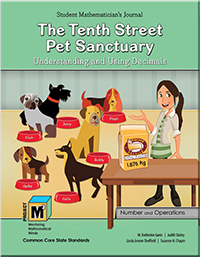# Project M³ Curriculum Units — Level 4-5

## The Tenth Street Pet Sanctuary: Understanding and Using Decimals

(get the book choose grades 4-5)Why is it that when we start to teach fractions and decimals, students sometimes struggle? Even talented students who can easily memorize and apply the rules sometimes do not understand the reasoning behind the rules. One reason is that the use of whole-number reasoning that students have spent so much time mastering often does not apply. For example, researchers Hiebert and Wearne (1986) found that when middle school students were asked which number is larger 0.059 or 0.2, the majority chose 0.059 since 59 was bigger than 2. Decimal operations also pose problems. As reported by the National Research Council (2001), a research study conducted with fifth, sixth, and seventh graders found that 68% of sixth graders and 51% of fifth and seventh graders stated that the sum of 4 + 0.3 was 0.7. These students were treating decimal numbers as whole numbers and did not even realize that the sum they found was actually less than one of the addends (4). The Common Core State Standards for Mathematics (2010) indicate the need for sufficient instructional time being dedicated to developing a conceptual understanding of decimals.

The Tenth Street Pet Sanctuary is an introduction to the decimal numeration system focusing on conceptual understanding and operations with decimals. Students explore decimals in the context of helping Peter and Petra operate a pet rescue center; measuring animal weights, food amounts, and costs. Experiences for the students include understanding how the powers of ten are used to create decimals and the role of the decimal point as the marker for the end of whole numbers, i.e., the ones place. Students also spend considerable time learning about the density property of the rational number system that states that between every two rational numbers, there is always another rational number.

In this unit, students also work with the four operations: addition, subtraction, multiplication, and division of decimals. The focus is on developing a strong understanding of the meaning of these operations with decimals. This involves understanding the context in a problem situation and constructing models and algorithms that make sense of the situations. It is important that students build the ability to conceptualize and interpret decimals beyond using money and not rely just on money to solve decimal problems. In this unit, we create base-ten models and use number line and area models, as well as discuss money applications.